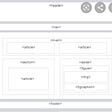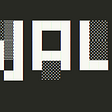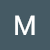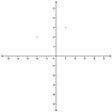# [Leet Code] Queries on a Permutation With Key

`Given the array queries of positive integers between 1 and m, you have to process all queries[i] (from i=0 to i=queries.length-1) according to the following rules:In the beginning, you have the permutation P=[1,2,3,...,m].For the current i, find the position of queries[i] in the permutation P (indexing from 0) and then move this at the beginning of the permutation P. Notice that the position of queries[i] in P is the result for queries[i].Return an array containing the result for the given queries.`
`Input: queries = [3,1,2,1], m = 5Output: [2,1,2,1] Explanation: The queries are processed as follow: For i=0: queries[i]=3, P=[1,2,3,4,5], position of 3 in P is 2, then we move 3 to the beginning of P resulting in P=[3,1,2,4,5]. For i=1: queries[i]=1, P=[3,1,2,4,5], position of 1 in P is 1, then we move 1 to the beginning of P resulting in P=[1,3,2,4,5]. For i=2: queries[i]=2, P=[1,3,2,4,5], position of 2 in P is 2, then we move 2 to the beginning of P resulting in P=[2,1,3,4,5]. For i=3: queries[i]=1, P=[2,1,3,4,5], position of 1 in P is 1, then we move 1 to the beginning of P resulting in P=[1,2,3,4,5]. Therefore, the array containing the result is [2,1,2,1].`
`class Solution(object):    def processQueries(self, queries, m):        """        :type queries: List[int]        :type m: int        :rtype: List[int]        """        answer = queries        p =  [number for number in range(1,m+1)]        for i, v in enumerate(queries):            answer[i] = p.index(v)            p.remove(v)            p.insert(0,v)        return answer`

--

--

--

Learning, and posting my findings!

Love podcasts or audiobooks? Learn on the go with our new app.

## SEMANTIC HTML AND ACCESSIBILITY## More Efficiently Health Kit with Awareness Kit## Search as you type in Flutter## This is my Architecture | Pipeline Architecture## CSS Grid Series —Recreating Codepen using CSS Grid (Part 1)## Write-up: HTB —Wall## AWS Lambda limitations## Matthew Boyd

Learning, and posting my findings!

## Identify Complete Binary Tree — Leetcode 958## [Leetcode] K Closest Points to Origin## DSA #4 Binary Tree and Binary Search Tree## Merge Sorted Array🐧